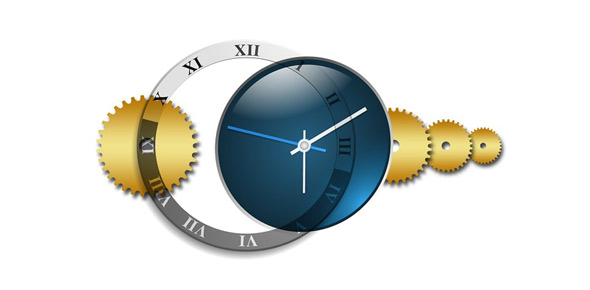# Can You Determine Right Time? Trivia Quiz

5 Questions | Attempts: 543
ShareSettings5 questions for Clock problems

• 1.
Find the number of degrees between the hands of a clock at 4:08
• A.

123 deg

• B.

76 deg

• C.

56 deg

• D.

24 deg

• 2.
Determine the angle in degrees between the clock of the hands at 3:45 PM
• A.

156 deg

• B.

158 deg

• C.

160 deg

• D.

162 deg

• 3.
Find the number of degrees between the hands of a clock at 4:32
• A.

123 deg

• B.

76 deg

• C.

56 deg

• D.

24 deg

• 4.
Find the two different times between 11 and 12 o'clock when the hands of the clock are at right angles to each other?
• A.

11:10 & 11:43

• B.

11:08 & 11:53

• C.

11:05 & 11:55

• D.

11:10 & 11:60

• 5.
The time is 5:24. The angle in degrees of the hour hand is:
• A.

162 deg

• B.

144 deg

• C.

152 deg

• D.

134 deg

## Related TopicsBack to top
×

Wait!
Here's an interesting quiz for you.